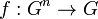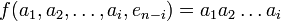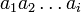# Equivalence of definitions of reducible multiary group

This article gives a proof/explanation of the equivalence of multiple definitions for the term reducible multiary group
View a complete list of pages giving proofs of equivalence of definitions

## Statement

Recall that a multiary group is a$n$-ary group for some$n \ge 2$. The following are equivalent for a$n$-ary group with multiplication$f:G^n \to G$:

1. There exists a group structure on$G$ such that$f(a_1,a_2,\dots,a_n) = a_1a_2\dots a_n$ for all (possibly repeated)$a_1,a_2,\dot,a_n \in G$, with the multiplication on the right being as per the group structure. For more on this, see group is n-ary group for all n
2. There exists a neutral element$e \in G$ for$f$:$f$ evaluated at any tuple where$n - 1$ of the entries are equal to$e$ and the remaining entry is$a \in G$, gives output$a$. This is true regardless of where we place$a$ and is also true if$a = e$.

## Proof

### (1) implies (2)

This is immediate: we can take$e$ to be the neutral element (i.e., the identity element) of$G$ as a group.

### (2) implies (1)

Given: A set$G$, function$f:G^n \to G$ making it a$n$-ary group, an element$e$ that is neutral for$f$.

To prove: There exists a group structure on$G$ with respect to which$f(a_1,a_2,\dots,a_n) = a_1a_2 \dots a_n$ for all$a_1,a_2,\dots,a_n \in G$.

Proof: We use the notation$e_i$ to denote$e$ written in succession$i$ times.

Step no. Assertion/construction Given data used Previous steps used Explanation
1$f(x,y,e_{n-2}) = f(e_i,x,e_j,y,e_k)$ for all$x,y \in G$ and all$i,j,k \ge 0$ with$i + j + k = n - 2$.$e$ is neutral for$f$,$f$ satisfies associativity becaus it gives a$n$-ary group operation By asociativity, we have$f(e_{k+1},f(e_i,x,e_j,y,e_k),e_{i+j}) = f(f(e_{k+1+i},x,e_j),y,e_{n-2})$. Using that$e$ is neutral, the outer$f$ of the left side vanishes and the left side becomes$f(e_i,x,e_j,y,e_k)$. On the right side, using that$f$ is neutral, the inside$f$ vanishes and we are left with$f(x,y,e_{n-2})$, completing the proof.
2 We can define an operation$xy := f(x,y,e_{n-2})$, and this equals$f$ evaluated on any tuple with$(n-2)$$e$s, one$x$ and one$y$, with the$x$ appearing to the left of the$y$. Step (1) Step-direct
3 The operation defined in Step (2) is associative, i.e.,$(ab)c = a(bc)$ for all$a,b,c \in G$$f$ satisfies$n$-ary associativity Step (2) We write the operation as$xy := f(x,e_{n-2},y)$. We thus get$(ab)c = f(f(a,e_{n-2},b),e_{n-2},c) = f(a,e_{n-2},f(b,e_{n-2},c)) = a(bc)$
4 The operation defined in Step (2) has identity element$e$$e$ is neutral for$f$ Step (2)$e$ works as an identity element: for all$a \in G$,$ae = f(a,e_{n-1}) = a$. Similarly,$ea = f(e_{n-1},a) = a$.
5 The operation defined in Step (2) admits two-sided inverses Steps (2), (3), (4) We show one-sided inverses from the unique solution condition: the right inverse of$a$ is the unique solution$b$ to$f(a,e,\dots,e,b) = e$. Similarly, the left inverse of$a$ is the unique$c$ such that$f(c,e,\dots,e,a) = a$. Now, we use the equality of left and right inverses in monoid to conclude that two-sided inverses exist.
6 The operation defined in Step (2) gives a group structure on$G$ Steps (2), (3), (4), (5) Step-combination direct
7 For any elements$a_1,a_2,\dots,a_i \in G$ with$i \le n$,$f(a_1,a_2,\dots,a_i,e_{n-i}) = a_1a_2\dots a_i$.$f$ is associative Steps (2), (6) We prove this by induction on$i$. Assuming true for$i - 1$, write$f(f(a_1,a_2,\dots,a_i,e_{n-i}),e_{n-1}) = f(a_1,f(a_2,\dots,a_i,e_{n-i+1})e_{n-2})$. The left side simplifies to the desired left side. On the right side, the second input simplifies to$a_2\dots a_i$ by inductive hypothesis, so now using the definition of group product, the product simplifies to$a_1a_2 \dots a_i$ as desired.
8 We are done Steps (2), (6), (7) By Steps (2) and (6), we have a group structure on$G$, which setting$i = n$ in Step (7), gives the desired$n$-ary group, completing the proof.

### Caution about non-uniqueness of neutral elements

For a reducible multiary group, there may be many different choices of neutral element. Each choice yields a potentially different binary operation, though the group structures we obtain are isomorphic. For instance, for the$n$-ary group obtained from the cyclic group of order$n - 1$, every element is a neutral element. The different group structures correspond to affine shifts in the original group, i.e., relabeling all elements by adding a group element to them.